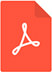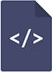# To square root the lagrangian or not: an underlying geometrical analysis on classical and relativistic mechanical models

Open Access Journal of Mathematical and Theoretical Physics
Rizzuti BF, Vasconcelos Júnior GF, Resende MAPDF Full Text

# Abstract

The geodesic has a fundamental role in physics and in mathematics: roughly speaking, it represents the curve that minimizes the arc length between two points on a manifold. We analyze a basic but misinterpreted difference between the Lagrangian that gives the arc length of a curve and the one that describes the motion of a free particle in curved space. They are taken as equivalent but it is not the case from the beginning. We explore this difference from a geometrical point of view, where we observe that the non-equivalence is nothing more than a matter of symmetry. The equivalence appears, however, after fixing a particular condition. As applications, some distinct models are studied. In particular, we explore the standard free relativistic particle, a couple of spinning particle models and also the forceless mechanics formulated by Hertz.

# Keywords

Hertz, Differential geometry, quantum phenomena, (semi-)riemannian manifold,second Newton law, classical mechanics, spinning particle